# Final

## Summary

We implemented bitwise neural networks on FPGA and run tests on the MNIST dataset. Experiments show that we achieve 4x speedup compared with the state-of-the-art FPGA implementation.

## Background

Deep neural networks (DNNs) have substantially pushed the state-of the-art in a wide range of tasks, including speech recognition and computer vision. However, DNNs also require a wealth of compute resources, and thus can benefit greatly from parallelization.

Moreover, power consumption recently has gained massive attentions due to the emerging of mobile devices. As is well known, running real-time text detection and recognition tasks on standalone devices, like a pair of glasses, will quickly drain the battery. Therefore, we need to exploit the heterogeneity of hardware to boost both performance and efficiency.

The attempt to implement neural networks on FPGA dates back to 24 years ago , yet no design shows a potential of commercial use until recently . However, the recent design still suffers from high resource usage and high latency. In this work, we propose a resource-efficient implementation, which also has higher throughput and lower latency.

## Contributions

Neural networks only have dependency between layers, and thus it is theoretically possible to fully parallelize inside a layer. However, due to millions, or even billions, of weights, this is practically impossible due to the lack of resources. In this project, we propose a novel implementation based on the bitwise neural networks. Specifically, we reschedule the compute process and successfully reuse some hardware components, which achieves a 1024-way parallelization with only a limited amount of resources.

## Bitwise Neural Networks

Kim et al. propose a resource efficient design of deep neural network , which replaces float multiplication with bit XNOR and float addition with bit counting. Specifically, we only need to do XNOR between inputs and weights in one layer and the output to next layer is activated if the counts of ‘1’s is greater than a threshold. By this change, the workload pattern becomes very suitable and highly parallelizable for hardware, e.g. FPGA, at the expense of only a small decrease of accuracy, from 98.9% to 98.7%.

## Optimizations

### Reduce the number of interconnections

In a fully-connected neural network, the number of weights grows O(n2) with a number of nodes. Our network has 1024 nodes per hidden layer, and this is equivalent to one million interconnections in hardware, which is certainly impractical.

Therefore, we want to reduce the number of interconnections. The intuition is that, instead of using a direct connection between node 1 and node 2 as shown in the left figure of Figure 1, we can use an indirect connection between node 1 and node 2 as in the right figure of Figure 1.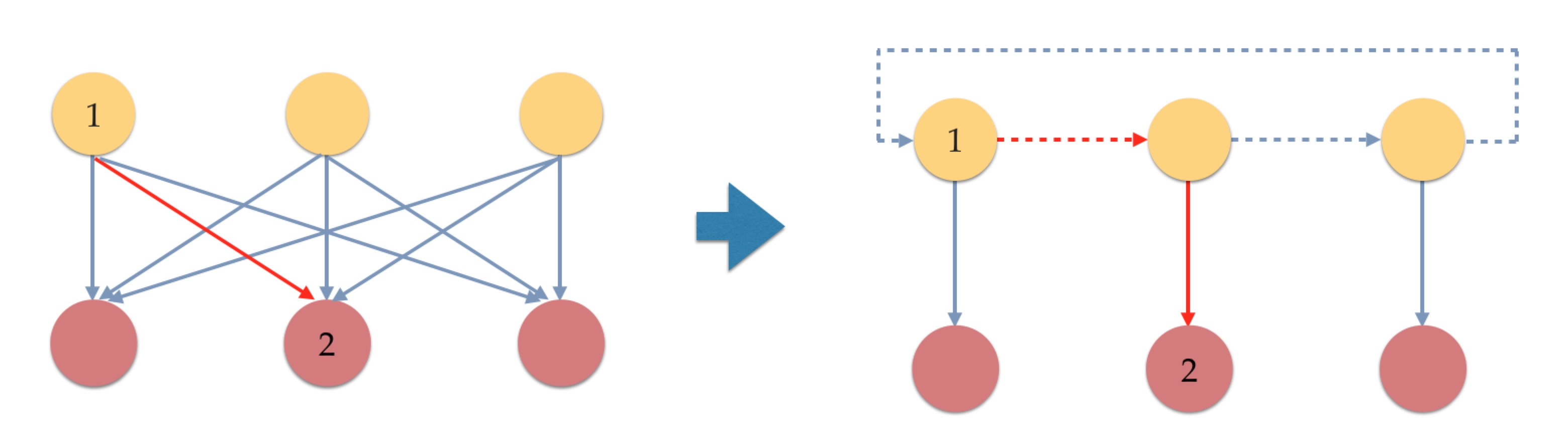##### Figure 1. Interconnections can be reused if we shift the inputs to proper locations

Concretely, one can simply express computation process between two layers with a matrix multiplication operation. Specifically, we first rearrange the contribution of each node due to commutative property as shown in Figure 2.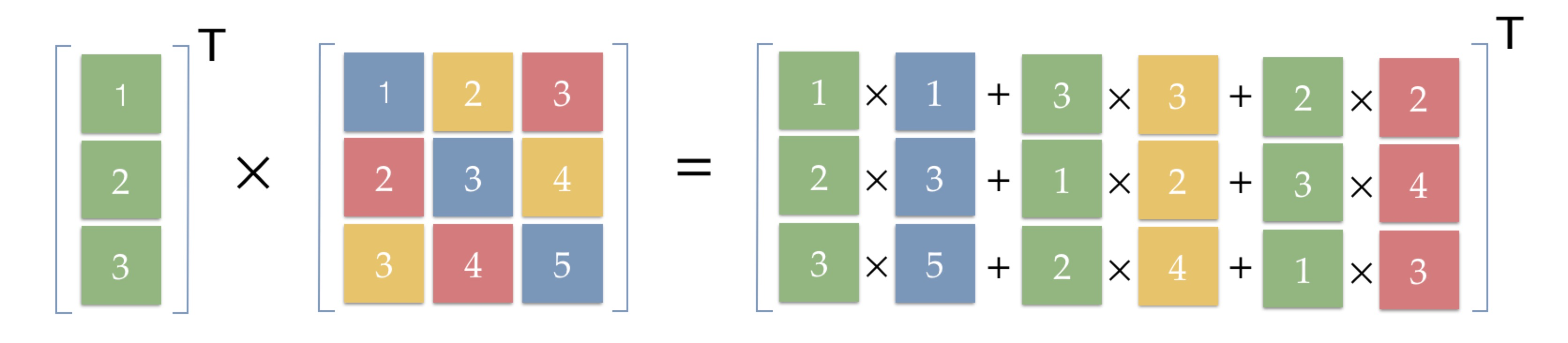##### Figure 2. Interconnections can be reused if we shift the inputs to proper locations

Note that in the result, the first green column is the initial input 1, 2 and 3, and the second green column is a shift of the first column. Also, the third column is a shift of the second column. Based on this updated computation order, we can obtain the final result of multiplication between a vector and an n2 matrix by n iterations as shown in Figure 3.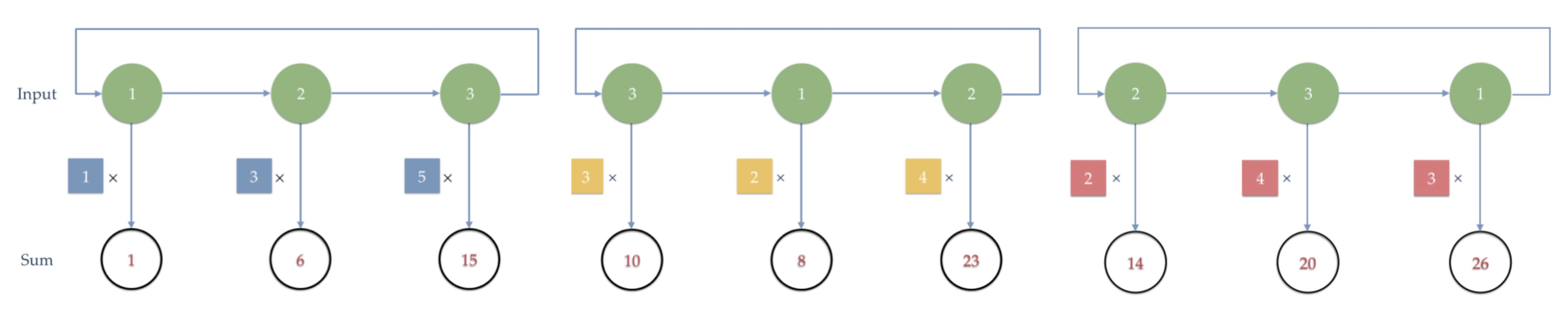##### Figure 3. Neural networks can be partitioned into n2 parts and each part contains only 1/n of the nodes

Therefore, if we rearrange the weights by diagonal, we can use shift and elementwise multiplication instead of matrix multiplication. By this means, we can reduce the interconnections from one million to two thousand for one layer, which is a 500 times saving. Also, the number of interconnections now grows only O(n) instead of O(n2) with the number of nodes, which is much more scalable.

### Matrix padding

Furthermore, the number of nodes in each layer is usually not the same. For example, we use a 784-1024-1024-1024-10 network. If we use different designs for different layers, it means 4 times more resources, and the resource usage for a deeper network will explode. The idea is to pad matrices that are less than 1024 x 1024 so that all layers can reuse the same hardware module. Considering that we have inactive weights in bitwise neural networks, we can use matrix padding for free. Specifically, an enable signal will decide whether the corresponding weight will count. By this means, we significantly save the hardware resources, in our scenario, by a factor of 4. A drawback is that we potentially may need to load more weights from memory, but the resource savings are more significant.

### Batch processing

It turns out that the program is bandwidth bound. To amortize the cost of loading, we can use batch processing. The idea is simple that we copy-and-paste the same module several times until we reach the resource limits. Each module shares the same set of weights from the memory, and therefore the loading cost is amortized.

### Implementation

Combining all ideas shown above, the final implementation is illustrated in Figure 4. It accepts binary inputs and gives binary outputs. It also contains XNOR module, enable signal, and bit counter. The activation, which is essentially a comparator, decides whether to activate the output based on the number of ‘1’s.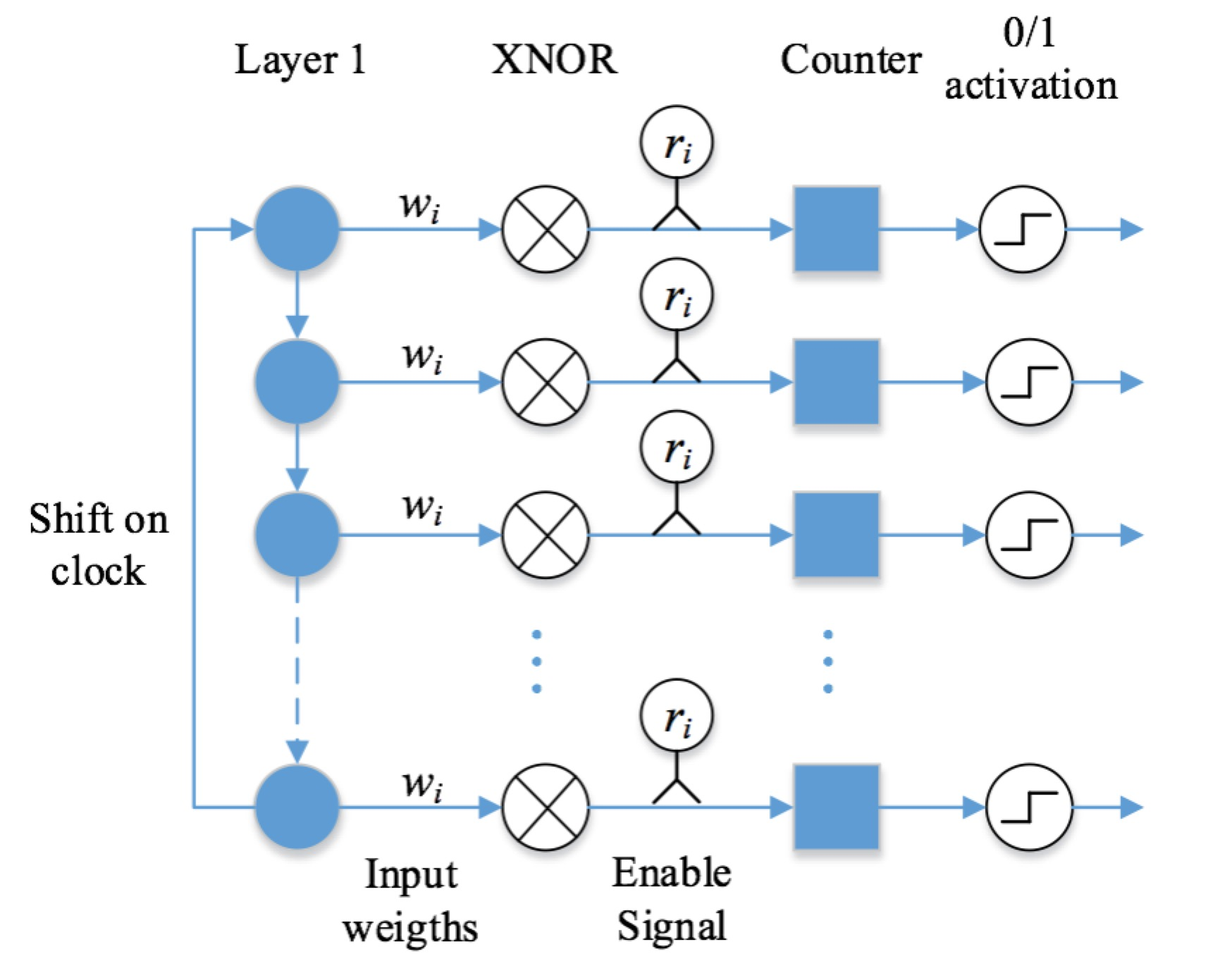## Evaluation

For evaluation, we target on the digit classification task using the MNIST dataset and all the following experiments are based on the Altera DE2-115 board. The board has 114,480 logic cells, 50 MHz clock and 2 MB SRAM.

In this section, we compare our design with the state-of-the-art approach, and we find that our approach greatly outperforms the state-of-the-art. Note that all measurements are converted to be resource-neural as the related paper tests on a much better FPGA than ours.

As shown in Figure 5, we speed up the classification phase by 1024x using our parallel algorithm. Compared with the cycles needed for loading weights, it is almost negligible. Then, we further use batch processing schemes to amortize the cost of loading, which reduces the loading cost by three times as we are able to use three modules in our hardware.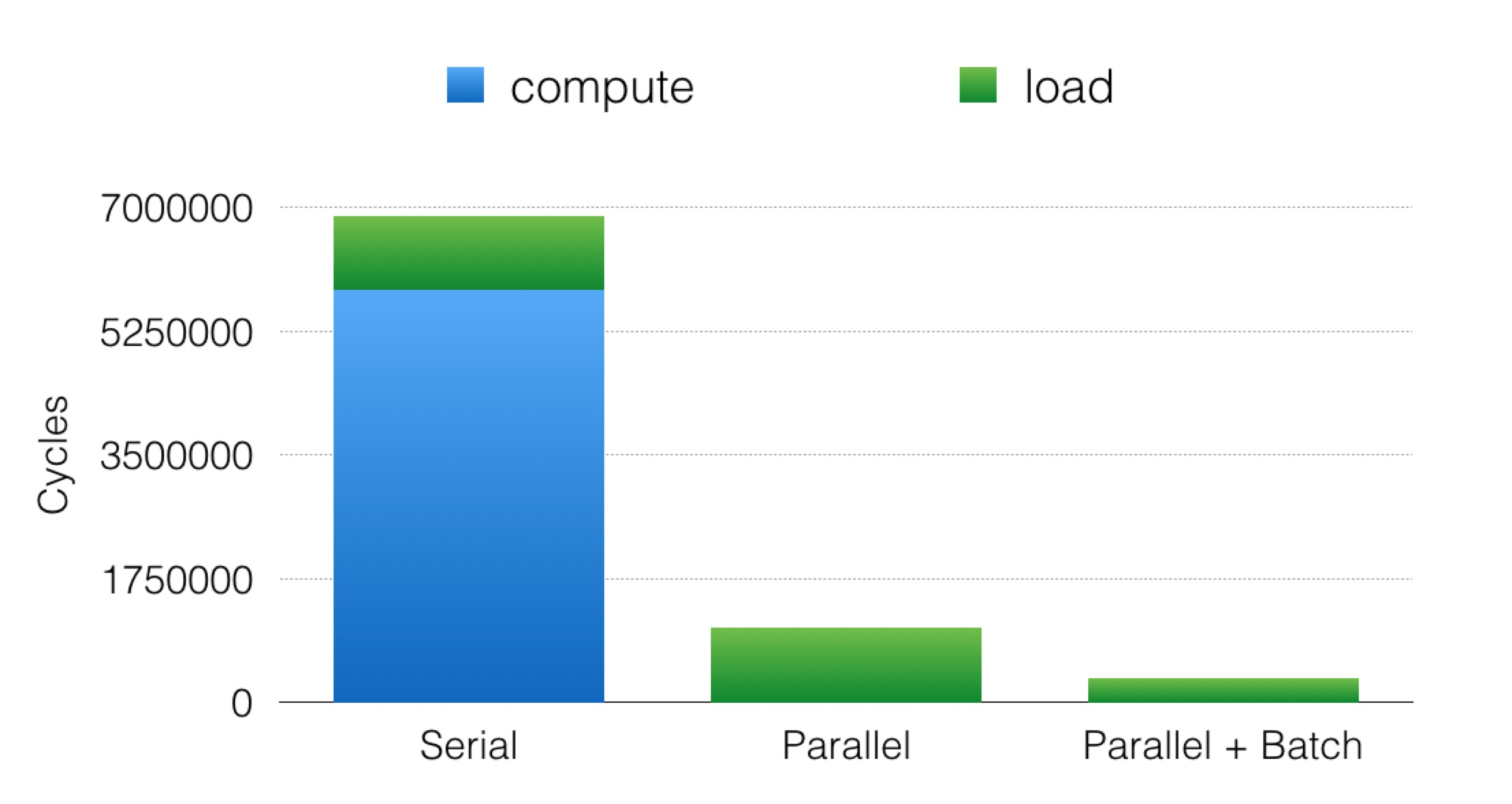##### Figure 5. The cycles needed for computing drop by a factor of 1024 and the loading cost can be amortized using batch processing

For comparison, we compare our paper with an existing paper that also implements FPGA to classify MNIST dataset. After compensating for the difference in the board, i.e. consider cycles rather than absolute time, our design achieve 4x throughput than .  Note that the throughput is normalized by the number of gates, i.e. they use over 210,000 logic cells, while we only use 33,000. Because we can always use batch processing to increase throughput if resources permit. Moreover, our design has a 60 times lower latency than , as they use a batch size of 100 to exchange latency for throughput. The real power measurement of  is 1 mJ per image, and the Altera power estimator shows our design consumes 0.56 mJ per image. Considering the possibility of under-estimating, the energy consumption could be very similar in the real world. A full comparison is shown in Figure 6.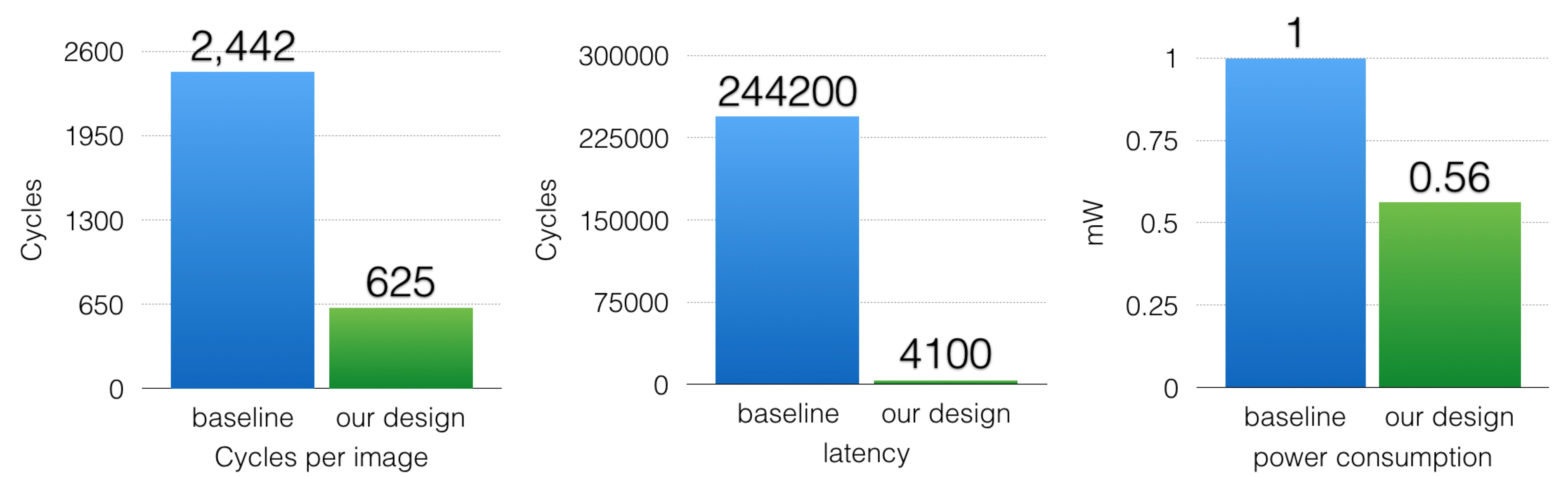##### Figure 6. Comparison of performance between our design and baseline FPGA paper

In the second part, we will compare our results with the open source tensorflow design running on a single-threaded CPU, as shown in Figure 7.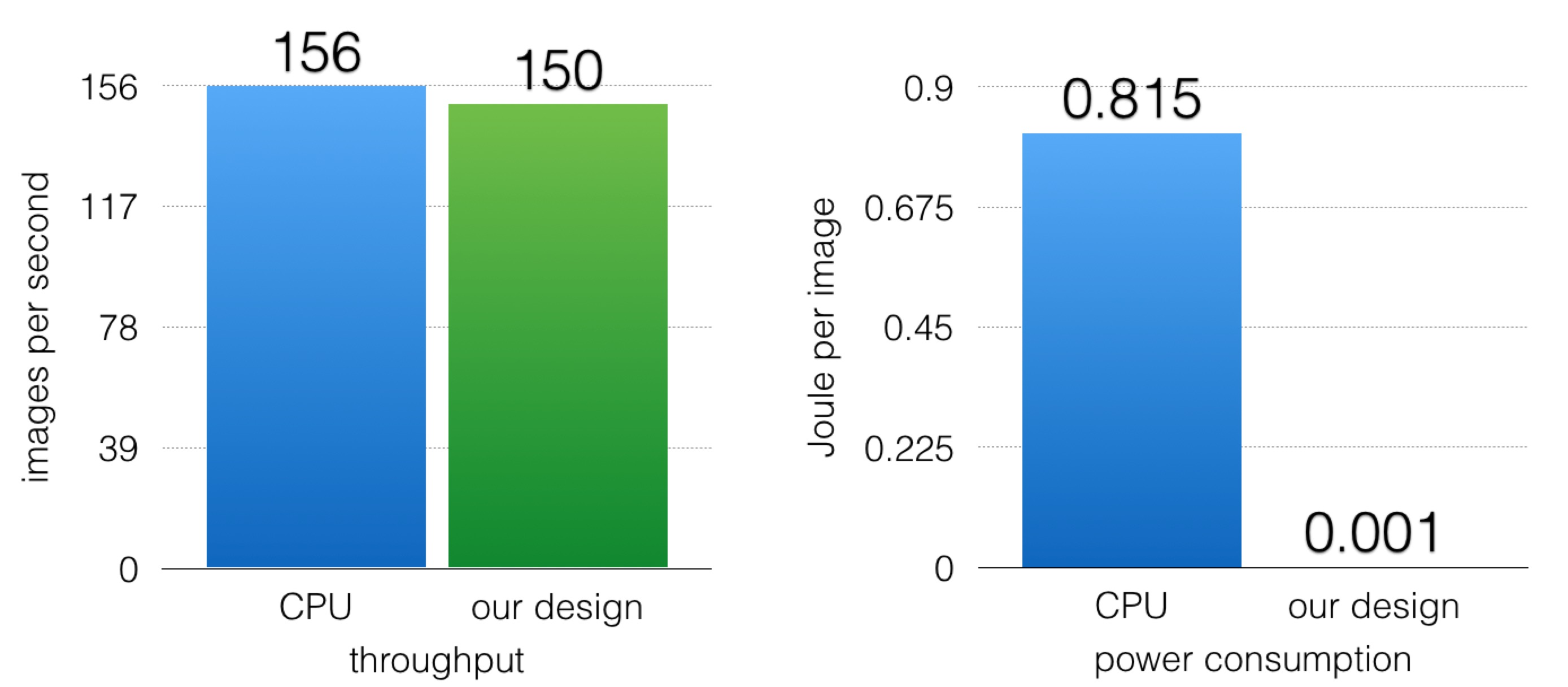##### Figure 7. Comparison of performance between our design and tensorflow on CPU

The CPU has 2.40GHz clock, while our board only has a 50 MHz clock. However, our throughput is very similar to the CPU throughput. Moreover, assuming that the CPU is loaded when executing digit recognition tasks, it will consume 127.1 Watt. Our board consumes 0.16 Watt. Therefore, our energy efficiency is 800 times better than the CPU design.

## Conclusions

FPGA implementation of neural networks benefit from higher parallelism, lower energy consumptions and no jitters. Our novel implementation of neural networks have a significant gain over the state-of-the-arts. Also, our design shows the potential of commercial use in more complex tasks.

## Acknowledgments

Thanks to 418 staffs for designing this wonderful project and bringing us to this interesting topic, especially Karima for proposing the initial idea. Also, thank Prof. Bill Nace for providing us with the hardware resources.

## References

•  Cox, C.E. and E. Blanz, GangLion - a fast field Programmable gate array implementation of a connectionist classifier. IEEE Journal of Solid-State Circuits, 1992. 28(3): p. 288-299.
•  Jinhwan Park, Wonyong Sung. Fpga Based Implementation of Deep Neural Networks Using On-chip Memory Only. arXiv preprint arXiv:1602.01616, 2016.
•  Minje Kim, and Paris Smaragdis. Bitwise Neural Networks. arXiv preprint arXiv:1601.06071v1, 2016

### Appendix I - Work Division

Equal work was performed by both project members.

### Appendix II - Personal Challenges

First, our implementation is based on a short paper from Kim et al. and we do not have any code available. Also, the paper does not record detailed information. Thus, we have to a lot of other papers and try a bunch of settings to reproduce the results.

Second, as non-ECE students, we did experience a hard time finding proper FPGA boards. Even after securing a board, we still encounter a lot of unexpected issues as we are non-ECE students. Due to the hardware problem, we have to make more backup plans, which brings troubles, but also help to shape our thoughts.

Third, as mentioned above, neural networks only have dependency between layers. Therefore, the first attempt tries to fully parallelize the entire layer by turning all weights to logical gates. Certainly, it is currently impossible to find one FPGA with at least 1024x1024 logic cells to store weights. Thus, we switched to our final design.

Along the way, we learn a wealth of new concepts and consistently change our plan according to the resources. In addition to the people mentioned in a previous section, we also sincerely thank everyone who have offered help or information, Kim, Darrin, etc.